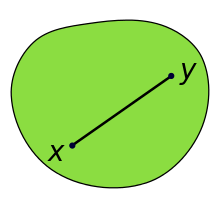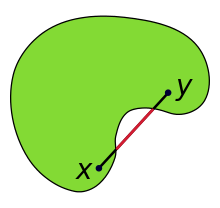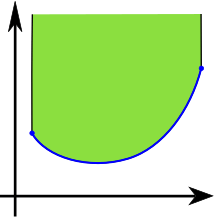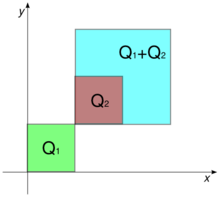# Convex setIllustration of a convex set which looks somewhat like a deformed circle. The (black) line segment joining points x and y lies completely within the (green) set. Since this is true for any points x and y within the set that we might choose, the set is convex.Illustration of a non-convex set. Since the red part of the (black and red) line-segment joining the points x and y lies outside of the (green) set, the set is non-convex.

In a Euclidean space (or, more generally in an affine space), a convex set is a region such that, for every pair of points within the region, every point on the straight line segment that joins the pair of points is also within the region. For example, a solid cube is a convex set, but anything that is hollow or has an indent, for example, a crescent shape, is not convex.

The boundary of a convex set is always a convex curve. The intersection of all convex sets containing a given subset A of Euclidean space is called the convex hull of A. It is the smallest convex set containing A.

A convex function is a real-valued function defined on an interval with the property that its epigraph (the set of points on or above the graph of the function) is a convex set. Convex minimization is a subfield of optimization that studies the problem of minimizing convex functions over convex sets. The branch of mathematics devoted to the study of properties of convex sets and convex functions is called convex analysis.

The notion of a convex set can be generalized to other spaces as described below.

## In vector spacesA function is convex if and only if its epigraph, the region (in green) above its graph (in blue), is a convex set.

Let S be a vector space over the real numbers, or, more generally, some ordered field. This includes Euclidean spaces. A set C in S is said to be convex if, for all x and y in C and all t in the interval [0, 1], the point (1 − t)x + ty also belongs to C. In other words, every point on the line segment connecting x and y is in C. This implies that a convex set in a real or complex topological vector space is path-connected, thus connected. Furthermore, C is strictly convex if every point on the line segment connecting x and y other than the endpoints is inside the interior of C.

A set C is called absolutely convex if it is convex and balanced.

The convex subsets of R (the set of real numbers) are simply the intervals of R. Some examples of convex subsets of the Euclidean plane are solid regular polygons, solid triangles, and intersections of solid triangles. Some examples of convex subsets of a Euclidean 3-dimensional space are the Archimedean solids and the Platonic solids. The Kepler-Poinsot polyhedra are examples of non-convex sets.

### Non-convex set

"Concave set" redirects here.

A set that is not convex is called a non-convex set. A polygon that is not a convex polygon is sometimes called a concave polygon, and some sources more generally use the term concave set to mean a non-convex set, but most authorities prohibit this usage.

The complement of a convex set, such as the epigraph of a concave function, is sometimes called a reverse convex set, especially in the context of mathematical optimization.

## Properties

If S is a convex set in n-dimensional space, then for any collection of r, r > 1, n-dimensional vectors u1, ..., ur in S, and for any nonnegative numbers λ1, ..., λr such that λ1 + ... + λr = 1, then one has:A vector of this type is known as a convex combination of u1, ..., ur.

### Intersections and unions

The collection of convex subsets of a vector space has the following properties:

1. The empty set and the whole vector-space are convex.
2. The intersection of any collection of convex sets is convex.
3. The union of a non-decreasing sequence of convex subsets is a convex set. For the preceding property of unions of non-decreasing sequences of convex sets, the restriction to nested sets is important: The union of two convex sets need not be convex.

### Closed convex sets

Closed convex sets are convex sets that contain all their limit points. They can be characterised as the intersections of closed half-spaces (sets of point in space that lie on and to one side of a hyperplane).

From what has just been said, it is clear that such intersections are convex, and they will also be closed sets. To prove the converse, i.e., every convex set may be represented as such intersection, one needs the supporting hyperplane theorem in the form that for a given closed convex set C and point P outside it, there is a closed half-space H that contains C and not P. The supporting hyperplane theorem is a special case of the Hahn–Banach theorem of functional analysis.

### Convex sets and rectangles

Let C be a convex body in the plane. We can inscribe a rectangle r in C such that a homothetic copy R of r is circumscribed about C. The positive homothety ratio is at most 2 and:## Convex hulls and Minkowski sums

### Convex hulls

Main article: convex hull

Every subset A of the vector space is contained within a smallest convex set (called the convex hull of A), namely the intersection of all convex sets containing A. The convex-hull operator Conv() has the characteristic properties of a hull operator:

 extensive S ⊆ Conv(S), non-decreasing S ⊆ T implies that Conv(S) ⊆ Conv(T), and idempotent Conv(Conv(S)) = Conv(S).

The convex-hull operation is needed for the set of convex sets to form a lattice, in which the "join" operation is the convex hull of the union of two convex sets

Conv(S) ∨ Conv(T) = Conv(S  T) = Conv(Conv(S)  Conv(T)).

The intersection of any collection of convex sets is itself convex, so the convex subsets of a (real or complex) vector space form a complete lattice.Minkowski addition of sets. The sum of the squares Q1=[0,1]2 and Q2=[1,2]2 is the square Q1+Q2=[1,3]2.

In a real vector-space, the Minkowski sum of two (non-empty) sets, S1 and S2, is defined to be the set S1 + S2 formed by the addition of vectors element-wise from the summand-sets

S1 + S2 = {x1 + x2 : x1  S1, x2  S2} .

More generally, the Minkowski sum of a finite family of (non-empty) sets Sn is the set formed by element-wise addition of vectorsFor Minkowski addition, the zero set {0} containing only the zero vector 0 has special importance: For every non-empty subset S of a vector space

S + {0} = S;

in algebraic terminology, {0} is the identity element of Minkowski addition (on the collection of non-empty sets).

### Convex hulls of Minkowski sums

Minkowski addition behaves well with respect to the operation of taking convex hulls, as shown by the following proposition:

Let S1, S2 be subsets of a real vector-space, the convex hull of their Minkowski sum is the Minkowski sum of their convex hulls

Conv(S1 + S2) = Conv(S1) + Conv(S2).

This result holds more generally for each finite collection of non-empty sets:In mathematical terminology, the operations of Minkowski summation and of forming convex hulls are commuting operations.

### Minkowski sums of convex sets

The Minkowski sum of two compact convex sets is compact. the sum of a compact convex set and a closed convex set is closed.

## Generalizations and extensions for convexity

The notion of convexity in the Euclidean space may be generalized by modifying the definition in some or other aspects. The common name "generalized convexity" is used, because the resulting objects retain certain properties of convex sets.

### Star-convex sets

Main article: Star domain

Let C be a set in a real or complex vector space. C is star convex if there exists an x0 in C such that the line segment from x0 to any point y in C is contained in C. Hence a non-empty convex set is always star-convex but a star-convex set is not always convex.

### Orthogonal convexity

An example of generalized convexity is orthogonal convexity.

A set S in the Euclidean space is called orthogonally convex or ortho-convex, if any segment parallel to any of the coordinate axes connecting two points of S lies totally within S. It is easy to prove that an intersection of any collection of orthoconvex sets is orthoconvex. Some other properties of convex sets are valid as well.

### Non-Euclidean geometry

The definition of a convex set and a convex hull extends naturally to geometries which are not Euclidean by defining a geodesically convex set to be one that contains the geodesics joining any two points in the set.

### Order topology

Convexity can be extended for a space X endowed with the order topology, using the total order < of the space.

Let YX. The subspace Y is a convex set if for each pair of points a, b in Y such that a < b, the interval (a, b) = {xX : a < x < b} is contained in Y. That is, Y is convex if and only if for all a, b in Y, a < b implies (a, b) ⊆ Y.

### Convexity spaces

The notion of convexity may be generalised to other objects, if certain properties of convexity are selected as axioms.

Given a set X, a convexity over X is a collection 𝒞 of subsets of X satisfying the following axioms:

1. The empty set and X are in 𝒞
2. The intersection of any collection from 𝒞 is in 𝒞.
3. The union of a chain (with respect to the inclusion relation) of elements of 𝒞 is in 𝒞.

The elements of 𝒞 are called convex sets and the pair (X, 𝒞) is called a convexity space. For the ordinary convexity, the first two axioms hold, and the third one is trivial.

For an alternative definition of abstract convexity, more suited to discrete geometry, see the convex geometries associated with antimatroids.

## References

1. McConnell, Jeffrey J. (2006), Computer Graphics: Theory Into Practice, p. 130, ISBN 0-7637-2250-2.
2. Takayama, Akira (1994), Analytical Methods in Economics, University of Michigan Press, p. 54, ISBN 9780472081356, An often seen confusion is a "concave set". Concave and convex functions designate certain classes of functions, not of sets, whereas a convex set designates a certain class of sets, and not a class of functions. A "concave set" confuses sets with functions.
3. Corbae, Dean; Stinchcombe, Maxwell B.; Zeman, Juraj (2009), An Introduction to Mathematical Analysis for Economic Theory and Econometrics, Princeton University Press, p. 347, ISBN 9781400833085, There is no such thing as a concave set.
4. Meyer, Robert (1970), "The validity of a family of optimization methods", SIAM Journal on Control and Optimization, 8: 41–54, MR 0312915.
5. Soltan, Valeriu, Introduction to the Axiomatic Theory of Convexity, Ştiinţa, Chişinău, 1984 (in Russian).
6. Singer, Ivan (1997). Abstract convex analysis. Canadian Mathematical Society series of monographs and advanced texts. New York: John Wiley & Sons, Inc. pp. xxii+491. ISBN 0-471-16015-6. MR 1461544.
7. Lassak, M. (1993). "Approximation of convex bodies by rectangles". Geometriae Dedicata. 47: 111. doi:10.1007/BF01263495.
8. The empty set is important in Minkowski addition, because the empty set annihilates every other subset: For every subset S of a vector space, its sum with the empty set is empty: S + ∅ = ∅.
9. Theorem 3 (pages 562–563): Krein, M.; Šmulian, V. (1940). "On regularly convex sets in the space conjugate to a Banach space". Annals of Mathematics (2), Second series. 41. pp. 556–583. doi:10.2307/1968735. JSTOR 1968735.
10. For the commutativity of Minkowski addition and convexification, see Theorem 1.1.2 (pages 2–3) in Schneider; this reference discusses much of the literature on the convex hulls of Minkowski sumsets in its "Chapter 3 Minkowski addition" (pages 126–196): Schneider, Rolf (1993). Convex bodies: The Brunn–Minkowski theory. Encyclopedia of mathematics and its applications. 44. Cambridge: Cambridge University Press. pp. xiv+490. ISBN 0-521-35220-7. MR 1216521.
11. Lemma 5.3: Aliprantis, C.D.; Border, K.C. (2006). Infinite Dimensional Analysis, A Hitchhiker's Guide. Berlin: Springer. ISBN 978-3-540-29587-7.
12. Rawlins G.J.E. and Wood D, "Ortho-convexity and its generalizations", in: Computational Morphology, 137-152. Elsevier, 1988.
13. Munkres, James; Topology, Prentice Hall; 2nd edition (December 28, 1999). ISBN 0-13-181629-2.
14. van De Vel, Marcel L. J. (1993). Theory of convex structures. North-Holland Mathematical Library. Amsterdam: North-Holland Publishing Co. pp. xvi+540. ISBN 0-444-81505-8. MR 1234493.Look up convex set in Wiktionary, the free dictionary.